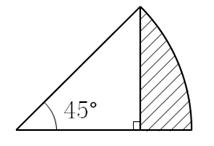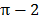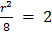### Sample Problem

If the area of the shaded region is, then what is the radius of the whole sector?

cm2#### Solution

The shaded region is the difference of the sector and the right isosceles triangle. Let’s assume the radius of the sector is r, so its area is.

If we draw the height of the triangle from the hypotenuse, the height is r/2. Thus, the area of the right triangle is.

Thus, the area is represented as.

Eliminatingfrom both sides of the equation, we have, so= 4 cm. We see that 42/4 also equals 4 so it works.